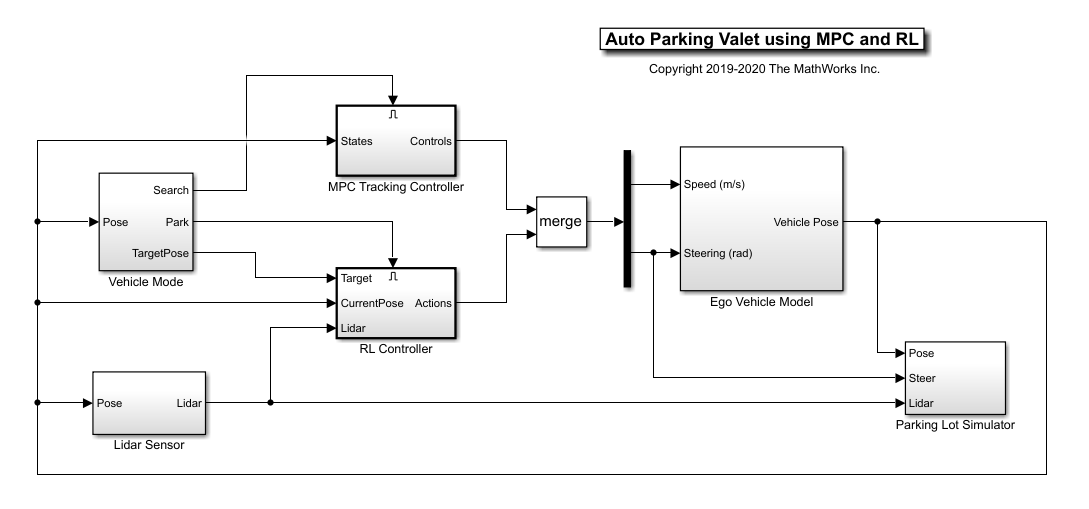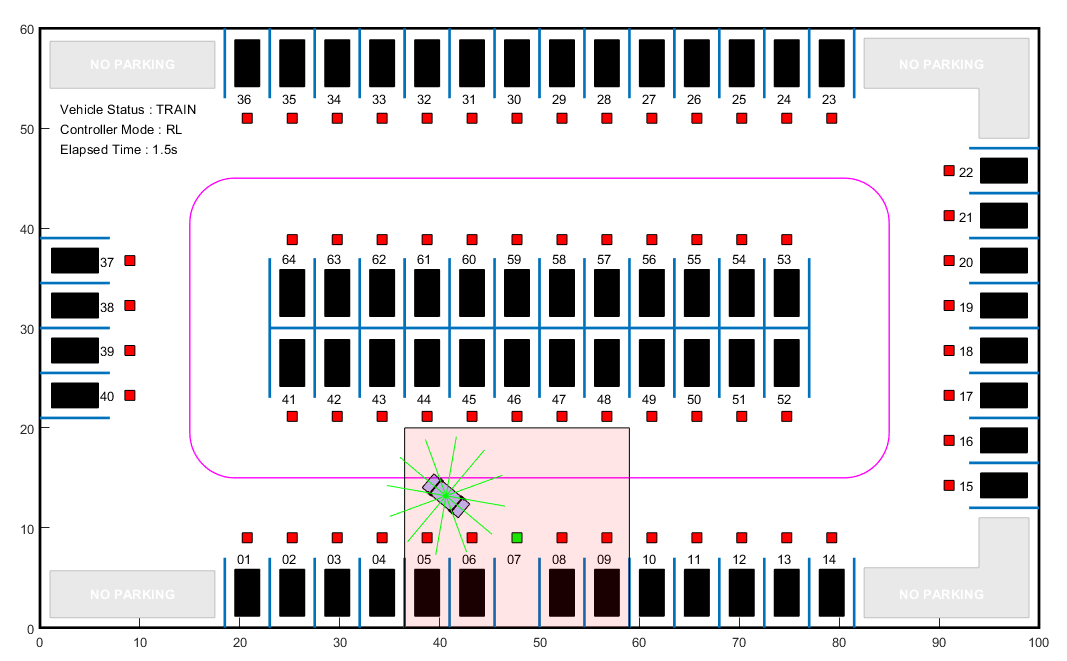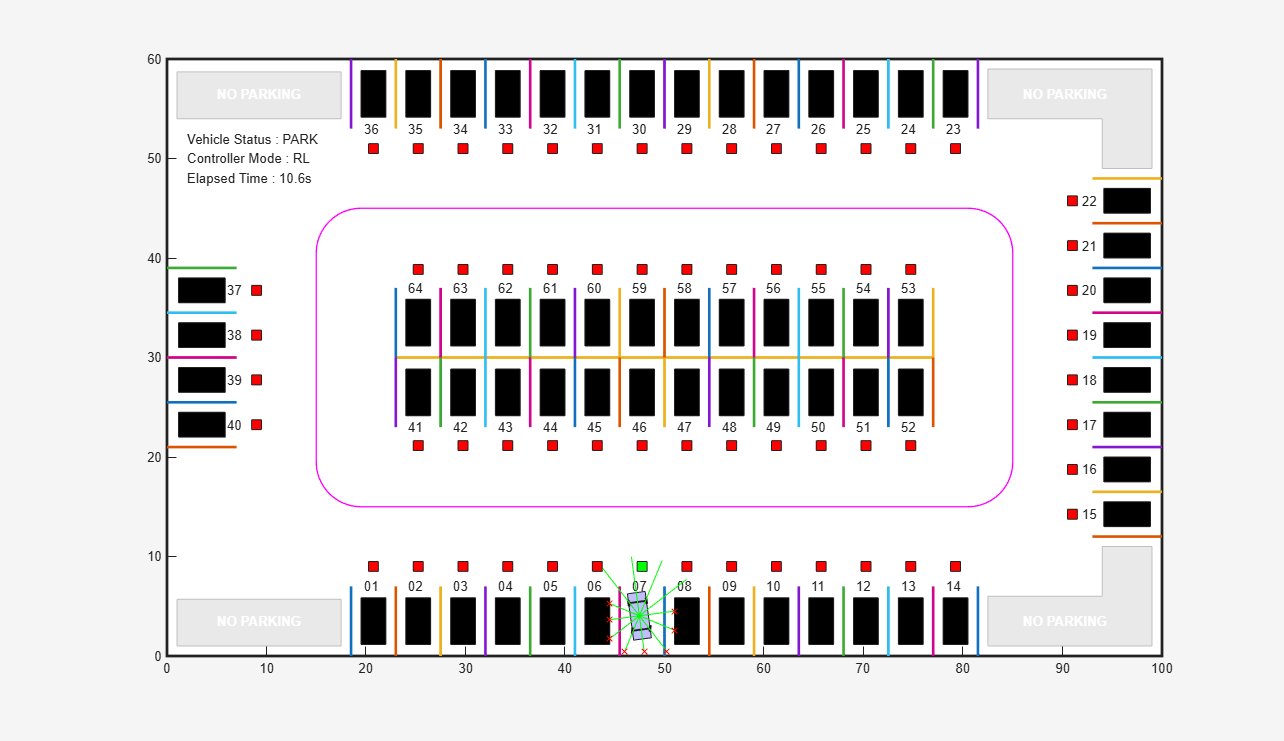# Train PPO Agent for Automatic Parking Valet

This example demonstrates the design of a hybrid controller for an automatic search and parking task. The hybrid controller uses model predictive control (MPC) to follow a reference path in a parking lot and a trained reinforcement learning (RL) agent to perform the parking maneuver.

The automatic parking algorithm in this example executes a series of maneuvers while simultaneously sensing and avoiding obstacles in tight spaces. It switches between an adaptive MPC controller and an RL agent to complete the parking maneuver. The MPC controller moves the vehicle at a constant speed along a reference path while an algorithm searches for an empty parking spot. When a spot is found, the RL Agent takes over and executes a pretrained parking maneuver. Prior knowledge of the environment (the parking lot) including the locations of the empty spots and parked vehicles is available to the controllers.

### Parking Lot

The parking lot is represented by the `ParkingLot` class, which stores information about the ego vehicle, empty parking spots, and static obstacles (parked cars). Each parking spot has a unique index number and an indicator light that is either green (free) or red (occupied). Parked vehicles are represented in black.

Create a `ParkingLot` object with a free spot at location 7.

```freeSpotIdx = 7; map = ParkingLot(freeSpotIdx);```Specify an initial pose $\left({\mathit{X}}_{0},{\mathit{Y}}_{0},{\theta }_{0}\right)$ for the ego vehicle. The target pose is determined based on the first available free spot as the vehicle navigates the parking lot.

`egoInitialPose = [20, 15, 0];`

Compute the target pose for the vehicle using the `createTargetPose` function. The target pose corresponds to the location in `freeSpotIdx`.

`egoTargetPose = createTargetPose(map,freeSpotIdx)`
```egoTargetPose = 1×3 47.7500 4.9000 -1.5708 ```

### Sensor Modules

The parking algorithm uses camera and lidar sensors to gather information from the environment.

#### Camera

The field of view of a camera mounted on the ego vehicle is represented by the area shaded in green in the following figure. The camera has a field of view $\phi$ bounded by $±60\text{\hspace{0.17em}}\mathrm{degrees}$ and a maximum measurement depth ${\mathit{d}}_{\mathrm{max}}$ of 10 m.As the ego vehicle moves forward, the camera module senses the parking spots that fall within the field of view and determines whether a spot is free or occupied. For simplicity, this action is implemented using geometrical relationships between the spot locations and the current vehicle pose. A parking spot is within the camera range if ${\mathit{d}}_{\mathit{i}}\le {\mathit{d}}_{\mathrm{max}}$ and ${\phi }_{\mathrm{min}}\le {\phi }_{\mathit{i}}\le {\phi }_{\mathrm{max}}$, where ${\mathit{d}}_{\mathit{i}}$ is the distance to the parking spot and ${\phi }_{\mathit{i}}$ is the angle to the parking spot.

#### Lidar

The reinforcement leangin agent uses lidar sensor readings to determine the proximity of the ego vehicle to other vehicles in the environment. The lidar sensor in this example is also modeled using geometrical relationships. Lidar distances are measured along 12 line segments that radially emerge from the center of the ego vehicle. When a lidar line intersects an obstacle, it returns the distance of the obstacle from the vehicle. The maximum measurable lidar distance along any line segment is 6 m.### Auto Parking Valet Model

The parking valet model, including the controllers, ego vehicle, sensors, and parking lot, is implemented in Simulink®.

Load the auto parking valet parameters.

`autoParkingValetParams`

```mdl = 'rlAutoParkingValet'; open_system(mdl)```The ego vehicle dynamics in this model are represented by a single-track bicycle model with two inputs: vehicle speed $\mathit{v}$ (m/s) and steering angle $\delta$ (radians). The MPC and RL controllers are placed within Enabled Subsystem blocks that are activated by signals representing whether the vehicle has to search for an empty spot or execute a parking maneuver. The enable signals are determined by the Camera algorithm within the Vehicle Mode subsystem. Initially, the vehicle is in search mode and the MPC controller tracks the reference path. When a free spot is found, park mode is activated and the RL agent executes the parking maneuver.

Create the adaptive MPC controller object for reference trajectory tracking using the `createMPCForParking` script. For more information on adaptive MPC, see Adaptive MPC (Model Predictive Control Toolbox).

`createMPCForParking`

### Reinforcement Learning Environment

The environment for training the RL agent is the region shaded in red in the following figure. Due to symmetry in the parking lot, training within this region is sufficient for the policy to adjust to other regions after applying appropriate coordinate transformations to the observations. Using this smaller training region significantly reduces training time compared to training over the entire parking lot.For this environment:

• The training region is a 22.5 m x 20 m space with the target spot at its horizontal center.

• The observations are the position errors ${\mathit{X}}_{\mathit{e}}$ and ${\mathit{Y}}_{\mathit{e}}$ of the ego vehicle with respect to the target pose, the sine and cosine of the true heading angle $\theta$, and the lidar sensor readings.

• The vehicle speed during parking is a constant 2 m/s.

• The action signals are discrete steering angles that range between +/- 45 degrees in steps of 15 degrees.

• The vehicle is considered parked if the errors with respect to target pose are within specified tolerances of +/- 0.75 m (position) and +/-10 degrees (orientation).

• The episode terminates if the ego vehicle goes out of the bounds of the training region, collides with an obstacle, or parks successfully.

• The reward ${\mathit{r}}_{\mathit{t}}$ provided at time t, is:

`${\mathit{r}}_{\mathit{t}}=2{\mathit{e}}^{-\left(0.05{{\mathit{X}}_{\mathit{e}}}^{2}+0.04{{\mathit{Y}}_{\mathit{e}}}^{2}\right)}+0.5{\mathit{e}}^{-40{{\theta }_{\mathit{e}}}^{2}}-0.05{\delta }^{2}+100{\mathit{f}}_{\mathit{t}}-50{\mathit{g}}_{\mathit{t}}$`

Here, ${\mathit{X}}_{\mathit{e}}$, ${\mathit{Y}}_{\mathit{e}}$, and ${\theta }_{\mathit{e}}$ are the position and heading angle errors of the ego vehicle from the target pose, and $\delta$ is the steering angle. ${\mathit{f}}_{\mathit{t}}$ (0 or 1) indicates whether the vehicle has parked and ${\mathit{g}}_{\mathit{t}}$ (0 or 1) indicates if the vehicle has collided with an obstacle at time $\mathit{t}$.

The coordinate transformations on vehicle pose $\left(\mathit{X},\mathit{Y},\theta \right)$ observations for different parking spot locations are as follows:

• 1-14: no transformation

• 15-22: $\stackrel{‾}{\mathit{X}}=\mathit{Y},\stackrel{‾}{\mathit{Y}}=-\mathit{X},\text{\hspace{0.17em}}\stackrel{‾}{\theta }=\theta -\pi /2$

• 23-36: $\stackrel{‾}{\mathit{X}}=100-\mathit{X},\stackrel{‾}{\mathit{Y}}=60-\mathit{Y},\text{\hspace{0.17em}}\stackrel{‾}{\theta }=\theta -\pi$

• 37-40: $\stackrel{‾}{\mathit{X}}=60-\mathit{Y},\stackrel{‾}{\mathit{Y}}=\mathit{X},\text{\hspace{0.17em}}\stackrel{‾}{\theta }=\theta -3\pi /2$

• 41-52: $\stackrel{‾}{\mathit{X}}=100-\mathit{X},\stackrel{‾}{\mathit{Y}}=30-\mathit{Y},\text{\hspace{0.17em}}\stackrel{‾}{\theta }=\theta +\pi$

• 53-64: $\stackrel{‾}{\mathit{X}}=\mathit{X},\stackrel{‾}{\mathit{Y}}=\mathit{Y}-28,\text{\hspace{0.17em}}\stackrel{‾}{\theta }=\theta$

Create the observation and action specifications for the environment.

```numObservations = 16; observationInfo = rlNumericSpec([numObservations 1]); observationInfo.Name = 'observations'; steerMax = pi/4; discreteSteerAngles = -steerMax : deg2rad(15) : steerMax; actionInfo = rlFiniteSetSpec(num2cell(discreteSteerAngles)); actionInfo.Name = 'actions'; numActions = numel(actionInfo.Elements);```

Create the Simulink environment interface, specifying the path to the RL Agent block.

```blk = [mdl '/RL Controller/RL Agent']; env = rlSimulinkEnv(mdl,blk,observationInfo,actionInfo);```

Specify a reset function for training. The `autoParkingValetResetFcn` function resets the initial pose of the ego vehicle to random values at the start of each episode.

`env.ResetFcn = @autoParkingValetResetFcn;`

For more information on creating Simulink environments, see `rlSimulinkEnv`.

### Create Agent

The RL agent in this example is a proximal policy optimization (PPO) agent with a discrete action space. PPO agents rely on actor and critic representations to learn the optimal policy. The agent maintains deep neural network-based function approximators for the actor and critic. To learn more about PPO agents, see Proximal Policy Optimization Agents.

Set the random seed generator for reproducibility.

`rng(0)`

To create the critic representations, first create a deep neural network with 16 inputs and one output. The output of the critic network is the state value function for a particular observation.

```criticNetwork = [ featureInputLayer(numObservations,'Normalization','none','Name','observations') fullyConnectedLayer(128,'Name','fc1') reluLayer('Name','relu1') fullyConnectedLayer(128,'Name','fc2') reluLayer('Name','relu2') fullyConnectedLayer(128,'Name','fc3') reluLayer('Name','relu3') fullyConnectedLayer(1,'Name','fc4')];```

Create the critic for the PPO agent using the . For more information, see `rlValueRepresentation` and `rlRepresentationOptions``.`

```criticOptions = rlRepresentationOptions('LearnRate',1e-3,'GradientThreshold',1); critic = rlValueRepresentation(criticNetwork,observationInfo,... 'Observation',{'observations'},criticOptions);```

The outputs of the actor networks are the probabilities of taking each possible steering action when the vehicle is in a certain state. Create the actor deep neural network.

```actorNetwork = [ featureInputLayer(numObservations,'Normalization','none','Name','observations') fullyConnectedLayer(128,'Name','fc1') reluLayer('Name','relu1') fullyConnectedLayer(128,'Name','fc2') reluLayer('Name','relu2') fullyConnectedLayer(numActions, 'Name', 'out') softmaxLayer('Name','actionProb')];```

Create a stochastic actor representation for the PPO agent. For more information, see `rlStochasticActorRepresentation`.

```actorOptions = rlRepresentationOptions('LearnRate',2e-4,'GradientThreshold',1); actor = rlStochasticActorRepresentation(actorNetwork,observationInfo,actionInfo,... 'Observation',{'observations'},actorOptions);```

Specify the agent options and create the PPO agent. For more information on PPO agent options, see `rlPPOAgentOptions`.

```agentOpts = rlPPOAgentOptions(... 'SampleTime',Ts,... 'ExperienceHorizon',200,... 'ClipFactor',0.2,... 'EntropyLossWeight',0.01,... 'MiniBatchSize',64,... 'NumEpoch',3,... 'AdvantageEstimateMethod',"gae",... 'GAEFactor',0.95,... 'DiscountFactor',0.998); agent = rlPPOAgent(actor,critic,agentOpts);```

During training, the agent collects experiences until it reaches experience horizon of 200 steps or the episode terminates and then trains from mini-batches of 64 experiences for three epochs. An objective function clip factor of 0.2 improves training stability and a discount factor value of 0.998 encourages long term rewards. Variance in critic the output is reduced by the generalized advantage estimate method with a GAE factor of 0.95.

### Train Agent

For this example, you train the agent for a maximum of 10000 episodes, with each episode lasting a maximum of 200 time steps. The training terminates when the maximum number of episodes is reached or the average reward over 100 episodes exceeds 100.

Specify the options for training using an `rlTrainingOptions` object.

```trainOpts = rlTrainingOptions(... 'MaxEpisodes',10000,... 'MaxStepsPerEpisode',200,... 'ScoreAveragingWindowLength',200,... 'Plots','training-progress',... 'StopTrainingCriteria','AverageReward',... 'StopTrainingValue',80);```

Train the agent using the `train` function. Training this agent is a computationally intensive process that takes several minutes to complete. To save time while running this example, load a pretrained agent by setting `doTraining` to `false`. To train the agent yourself, set `doTraining` to `true`.

```doTraining = false; if doTraining trainingStats = train(agent,env,trainOpts); else load('rlAutoParkingValetAgent.mat','agent'); end```### Simulate Agent

Simulate the model to park the vehicle in the free parking spot. To simulate the vehicle parking in different locations, change the free spot location in the following code.

```freeSpotIdx = 7; % free spot location sim(mdl);```The vehicle reaches the target pose within the specified error tolerances of +/- 0.75 m (position) and +/-10 degrees (orientation).

To view the ego vehicle position and orientation, open the Ego Vehicle Pose scope.